Over 95% of employees think Income Tax is far too complicated. Calculate your own income tax with data from your payslip and learn to calculate yourself!

## First, Open the Income Tax Calculator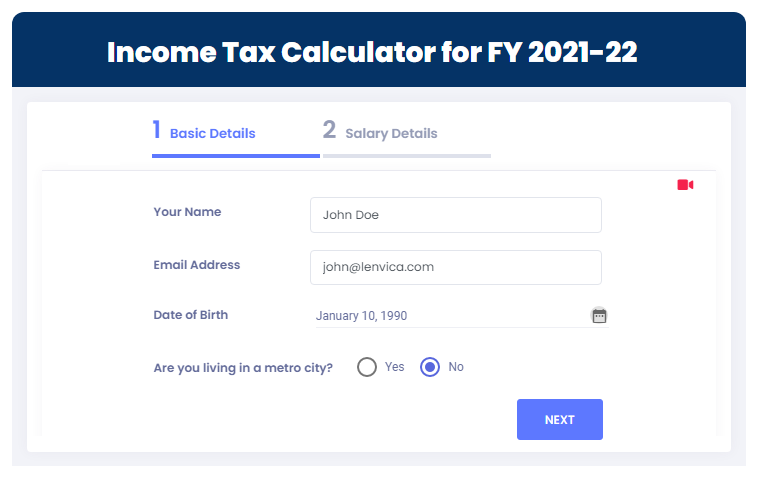## Key in data from your payslip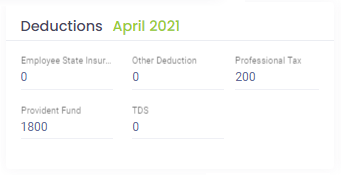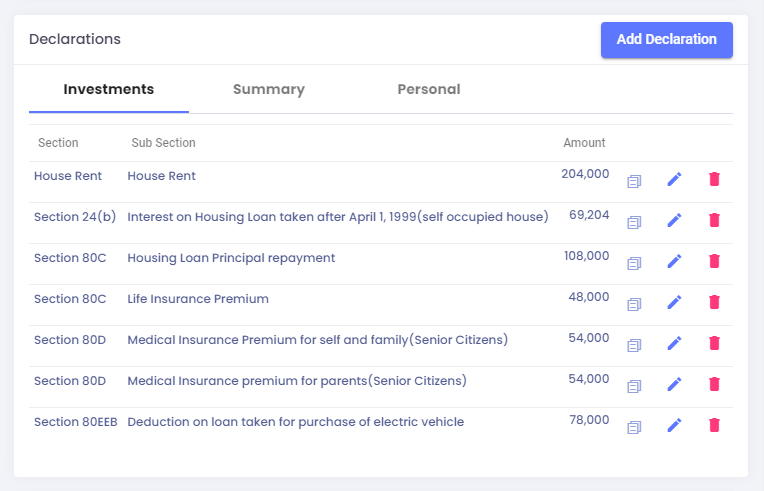## Calculate Income Tax !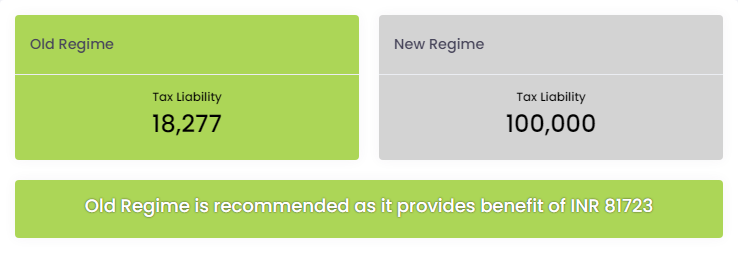Congratulations! You have calculated your own Income tax. Now, let’s learn how it is calculated.

Let’s go to the Calculation Summary and learn the 4 steps to calculate Income Tax.

## Step 1: Calculate HRA Exemption

HRA exemption is calculated as the least of D, E and F shown in table below.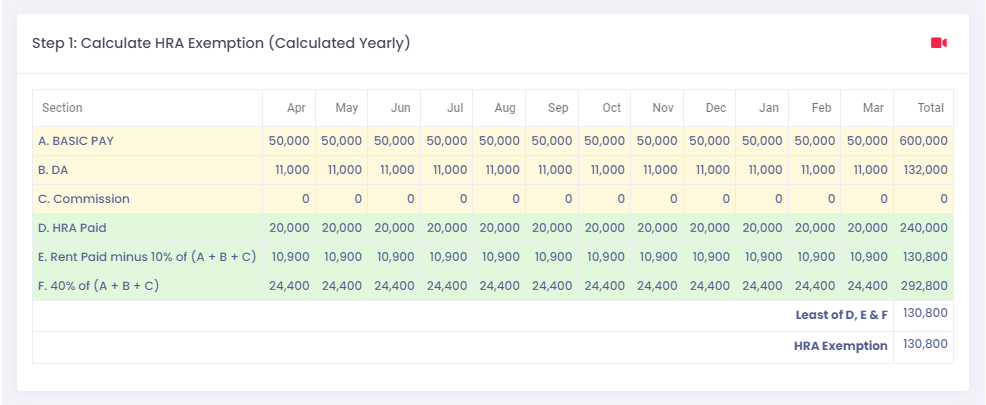Where D is the HRA paid by your employer.
E is the rent paid over 10% of (Basic Pay + DA + Commission).
And F is 40% of (Basic Pay + DA + Commission).

To learn this in detail, watch the help video below!

## Step 2: Calculate Taxable Income

In New Regime, Taxable Income is calculated as (Gross Salary + Bonus + Other Income – Deductions under Chapter VI A).

The Old regime calculates taxable income with a similar formula which also includes more deductions, exemptions and a standard deduction.To learn in detail, watch the video below!

## Step 3: Calculate Income tax by applying Taxable Income on Tax slab

The New Regime has a fixed tax slab as shown.
The Old Regime has a varying slab according to the age of the employee.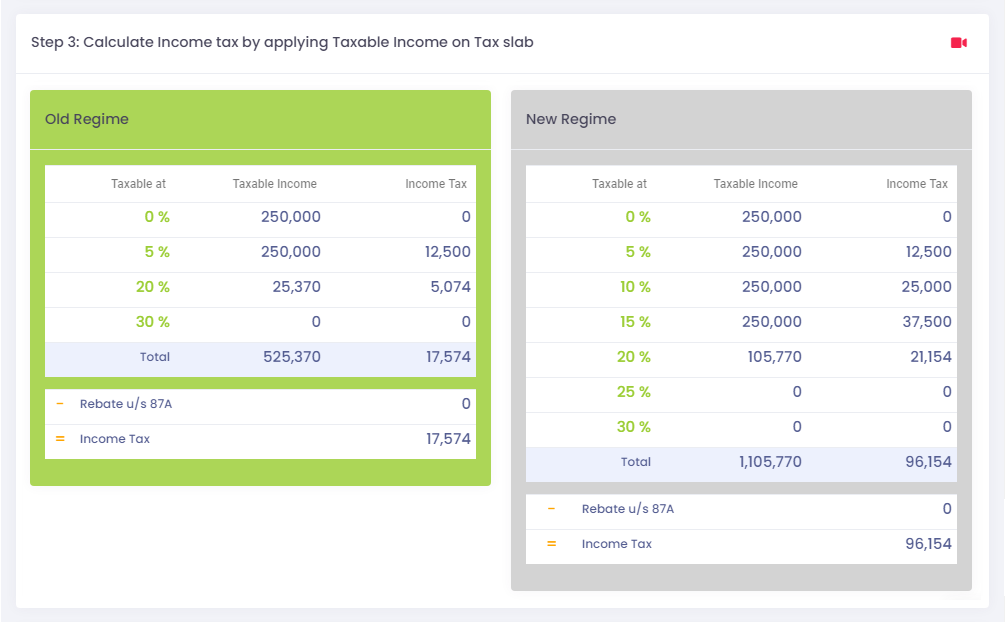To learn in detail, watch the video below!

## Step 4: Calculate Tax Liability

The total tax liability is calculated in the same way for both Old and New Regime. Tax Liability is calculated as sum of Income Tax calculated in the previous step, Surcharge on the income tax calculated, and the Health and Education Cess imposed by the Government.To learn in detail, watch the help video available here!

The Income Tax Calculator calculates Income Tax from your payslip and also explains how it is calculated. Just experiment with the Income Tax Calculator to learn and understand Income Tax in no time.